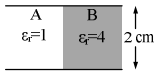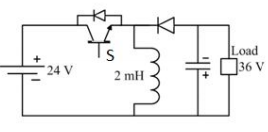# GATE 2016 Electrical Solution/Key.pdf

Q.16 A parallel plate capacitor filled with two dielectrics is shown in the figure below. If the electric field in the region A is 4 kV/cm, the electric field in the region B, in kV/cm, is(A) 1
(B) 2
(C) 4
(D) 16

Q.17 A 50 MVA, 10 kV, 50 Hz, star-connected, unloaded three-phase alternator has a synchronous reactance of 1 p.u. and a sub-transient reactance of 0.2 p.u. If a 3-phase short circuit occurs close to the generator terminals, the ratio of initial and final values of the sinusoidal component of the short circuit current is ________.

Q.18 Consider a linear time-invariant system with transfer function
H(s) = 1/(1+s)
If the input is cos(t) and the steady state output is A cos(t+ a), then the value of A is _________.

Q.19 A three-phase diode bridge rectifier is feeding a constant DC current of 100 A to a highly inductive load. If three-phase, 415 V, 50 Hz AC source is supplying to this bridge rectifier then the rms value of the current in each diode, in ampere, is _____________.
Q.20 A buck-boost DC-DC converter, shown in the figure below, is used to convert 24 V battery voltage to 36 V DC voltage to feed a load of 72 W. It is operated at 20 kHz with an inductor of 2 mH and output capacitor of 1000 µF. All devices are considered to be ideal. The peak voltage across the solid-state switch (s) in volts, is _Please report if you find any errors in the questions.
Answers/Solution of remaining questions are under evaluation and the same will be published here soon.
Keep watching the spaceThanks for reading GATE 2016 Electrical Solution/Key.pdf

←Previous
« Prev Post
Next→
Next Post »